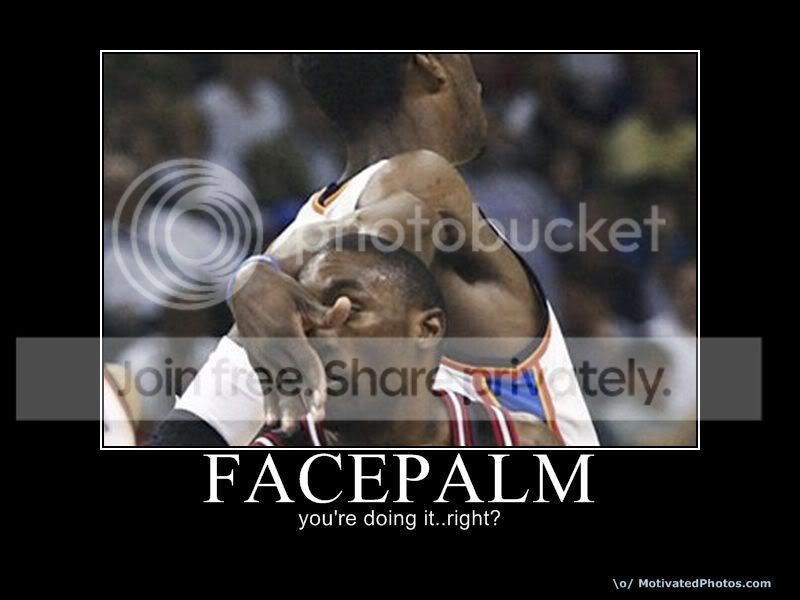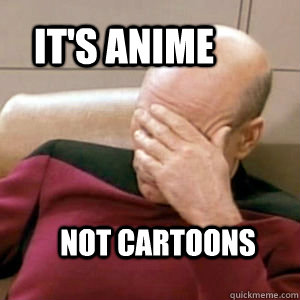# The Official Forum of Wandering Minds

280 posts
Originally posted by sharkdog123 :lAKATSUKIl:A. sorry
B. i don't know who eather of them are(please don't face palm)
http://www.glassgiant.com/ascii/ascii.php
Sorry, this file could not be opened. Possible reasons:

The file size may be greater that 3 Mb. The image you send must be less than that.

If none of these steps resolves the problem, you could always try saving the image to your computer and using the "From Your Computer" option. This can usually be done by right-clicking on the image and clicking Save or Save As...
^^^^^^^^^^^^^^^^^^^^^^^^^^^^^^^^^^^^^^^^^^^^^^^^^^^^^
^^^^^^^^^^^^^^^^^^^^^^^^^^^^^^^^^^^^^^^^^^^^^^^^^^^^^
^^^^^^^^^^^^^^^^^^^^^^^^^^^^^^^^^^^^^^^^^^^^^^^^^^^^^
^^^^^^^^^^^^^^^^^^^^^^^^^^^^^^^^^^^^^^^^^^^^^^^^^^^^^
^^^^^^^^^^^^^^^^^^^^^^^^^^^^^^^^^^^^^^^^^^^^^^^^^^^^^
^^^^^^^^^^^^^^^^^^^^^^^^^^^^^^^^^^^^^^^^^^^^^^^^^^^^^
^^^^^^^^^^^^^^^^^^^^^^^^^^^^^^^^^^^^^^^^^^^^^^^^^^^^^
^^^^^^^^^^^^^^^^^^^^^^^^^^^^^^^^^^^^^^^^^^^^^^^^^^^^^
^^^^^^^^^^^^^^^^^^^^^^^^^^^^^^^^^^^^^^^^^^^^^^^^^^^^^
^^^^^^^^^^^^^^^^^^^^^^^^^^^^^^^^^^^^^^^^^^^^^^^^^^^^^
^^^^^^^^^^^^^^^^^^^^^^^^^^^^^^^^^^^^^^^^^^^^^^^^^^^^^
^^^^^^^^^^^^^^^^^^^^^^^^^^^^^^^^^^^^^^^^^^^^^^^^^^^^^
^^^^^^^^^^^^^^^^^^^^^^^^^^^^^^^^^^^^^^^^^^^^^^^^^^^^^
^^^^^^^^^^^^^^^^^^^^^^^^^^^^^^^^^^^^^^^^^^^^^^^^^^^^^
That is what happen when I click the link. It said that to me in really really small text.
Hakuna Matata, because it just seems like a total stoner wrote it.
What???
Originally posted by sharkdog123 :lAKATSUKIl:What???
IT ISN'T A PERSON! IT IS A SONG!!! havent you ever seen lion king?
haven't you heard it? it's about being mellow, and having no worries, ergo, stoner, or Buddhist
A. I don't have a religion.
B. You sound a lot like one of my friends who is always telling me to stop stressing.
C.Well everybody's heard about the bird !
Bird bird bird, the bird is the word !

Everybody's heard about the bird !
Bird bird bird, the bird is the word !

Don't you know about the bird?
Well everybody's heard about the bird !
um, wtf?
mu, ftw?
Shizzle.
Elzzihs.
Da Nizzle
Originally posted by sharkdog123 :lAKATSUKIl:A. sorry
B. i don't know who eather of them are(please don't face palm)
*facepalm*
Originally posted by Mosta Fox
Originally posted by sharkdog123 :lAKATSUKIl:A. sorry
B. i don't know who eather of them are(please don't face palm)
*facepalm*
*facepalm* because he facepalmed
Originally posted by (L4D2)Karmacharger110
Originally posted by Mosta Fox
Originally posted by sharkdog123 :lAKATSUKIl:A. sorry
B. i don't know who eather of them are(please don't face palm)
*facepalm*
*facepalm* because he facepalmed
*Facepalms so hard it rips through my face.* AAAAAAAAAH!!!!
Facepalm has overwhelmed my forum. Quick! Who can post the best facepalm?Rwhite wins! For being the most literal is his interpretation of a facepalm!
Not yet. Don't direct me forum.
I can't do faceplams or i will have my hands removed from this forum as well
Originally posted by MetropalNot yet. Don't direct me forum.
Well he has the funniest. . .
Bu there have only been two.
Exactly. MOAR!!!!!!!!!!
am I aloud?
Originally posted by I.I.C.D.M.M Sauron |FBG\$|Bu there have only been two.
I DON'T SEE ANYONE ELSE POSTING!!!!!!!
AM I ALOUD TOO?
Allowed*

And why not? Post what you want. It's not exactly like I can stop you.
You are allowed to post facepalms in this out of context situation, shark. No hands will be removed.
ok goodnova
Originally posted by CrossingOblivionForgive the rude question that follows, but who makes the sandwiches in lesbian relationship?
Well, the........uh........
Lol.
any of them good?
Pokemon one is decent.
yay
I like the one with the hand through the face
Thank youbetter
thanksOriginally posted by sharkdog123 :lAKATSUKIl:i like this one! lollz
Meh. I agree that the tree is the best so far.